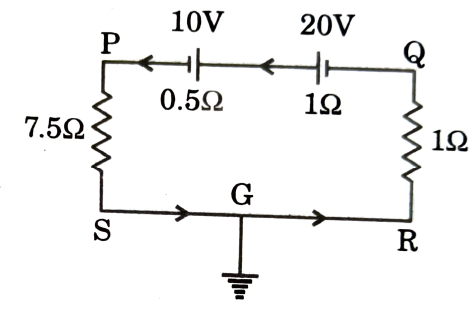Sources of emf and Kirchhoff's laws
Question

# In the circuit shown:Moderate
Solution

## The current in the circuit I $=\frac{\left(20-10\right)}{\left(7.5+0.5+1+1\right)}$                                          $=\frac{10}{10}=1\mathrm{amp}$The potential difference across $\mathrm{PS},{\mathrm{V}}_{\mathrm{PS}}=7.5×1$                                                               $=7.5\mathrm{V}$The potential difference across $\mathrm{Q},\mathrm{R},{\mathrm{V}}_{\mathrm{QR}}=1×1$                                                                  $=1\mathrm{V}$As point G is connected to earth, hence potentials of R and S are zero.The direction of the current in the circuit is from P to S, hence point P is at higher potential.$\therefore$Hence (b), (c) and (d) are correct.

Get Instant Solutions